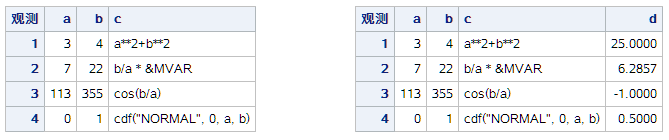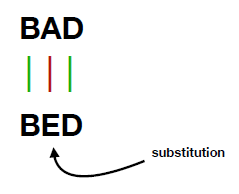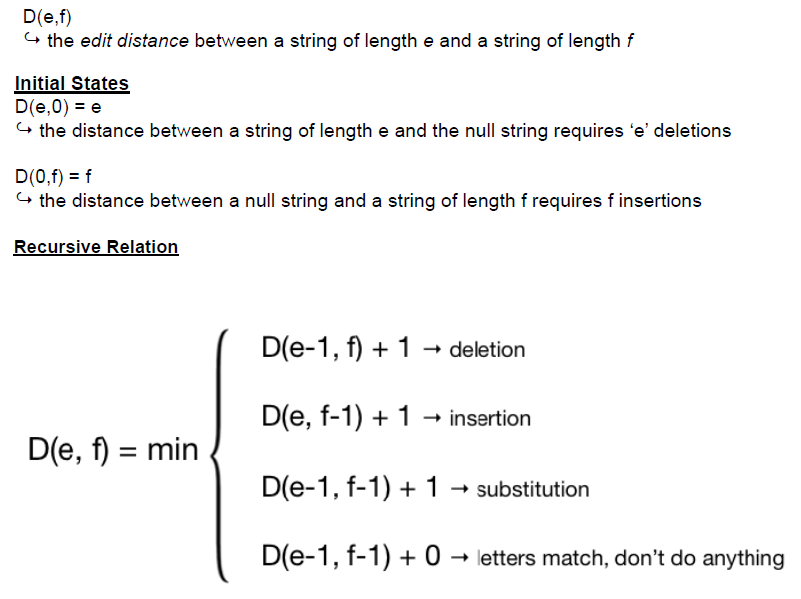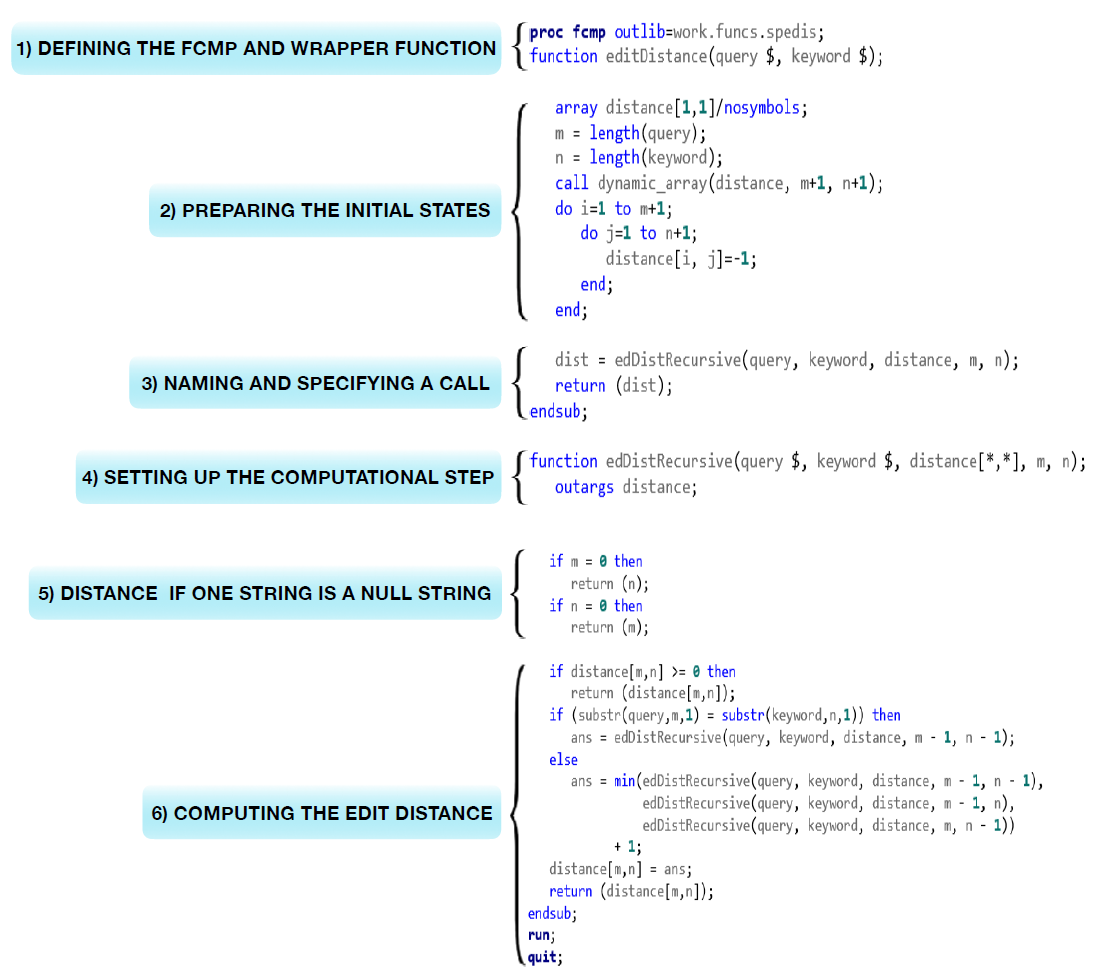In many programming languages, there is a function named eval() that can be used to evaluate an expression and return the result at run time. For example, in Python, the eval() function parses the expression passed to it and runs a Python expression or code within the program. Even Python eval() supports more optional parameters, such as global/local dictionary for runtime context, but the goal for evaluation remains the same.

When an expression is stored as a character variable of an SAS observation, it suggests the running context is the SAS program execution phase, so the internal data structure like Input Buffer (IB), Program Data Vectors (PDV) and Descriptor Information of the output database are all accessible. Furthermore, all SAS system functions are also accessible in an expression, just like the raw SAS code exposed to SAS compiler and runtime. In the following SAS data set, what the user wants is to get the real value from the expression in variable C. It is different from the commonly used Calculated Column, which uses the same computing rule to expand a new variable for all observations. Here the column C has a different computing rule for each observation; the user's expected result is the column D.

 %let MVAR=2; data a; length a b 8 c \$ 255; a=3; b=4; c='a**2+b**2'; output; /* Arithmetic or Logical */ a=7; b=22; c='b/a * &MVAR'; output; /* SAS Macro Var*/ a=113; b=355; c='cos(b/a)'; output; /* Trigonometric */ a=0; b=1; c='cdf("NORMAL", 0, a, b)'; output; /* Probability */ run; proc print;run;Someone might want a solution that parses the expression in variable C, and then try to rebuild the abstract syntax tree and try to evaluate it from bottom to top. I can’t say this solution is totally wrong but it’s very complex and too hard to generate a general solution. If we have an SAS function eval() in DATA Step, then we can easily use the following code to achieve the goal. Unfortunately, SAS did not provide the eval() function.

 data b; set a; d=eval(c); run;

SAS provides Macro function %eval, %sysevalf to evaluate arithmetic and logical expressions using integer or floating-point arithmetic. SAS also provides function resolve() (not supported in the DATA Step that runs in CAS) to return the resolved value of the argument after it has been processed by the macro facility. Anyway, resolve() can’t access the values of macro variable assigned by symput() or symputn() at program execution phase. So, for the SAS code as below, it outputs c=150 and d=200. For simple arithmetic and logical expression, we can use the tranwrd() function to replace all variable names with real values, and then use resolve('%sysevalf('|| cats(expression_with_real_values) || ')') to get evaluated result, but it limited to only SAS functions supported by %eval() and %sysevalf() macro functions.

 %let a=100; %let b=50; data _null_; call symput("b", 200); c=resolve("%eval(&a+&b)"); put c=; d=symget("b"); put d=; run;

Now let’s return to the key question we raised: How can we implement the eval() function in SAS Data Step? The best solution we found so far is to use the dynamic code generation mechanism in SAS, and then submit the code to SAS for parsing and execution. In this way, we retrieve string expression from a variable to a real expression in SAS code successfully. We don’t care what the valid SAS expression is, we totally convert it to the code snippets and submit it for execution. Syntax check, reference to PDV variables, internal functions, and even SAS Macro variables are all supported. Yes, it’s a smart and concise implementation for general purposes.

Due to each observation having a different computing rule, we need to use _N_ to control the observation mapping. So, for the existing dataset A, we can use the following code to achieve the goal. The key secret is to use CALL EXECUTE to generate SAS code dynamically and delay execution after the code is ready. In the output dataset B, we have a numeric column D with the value evaluated with the character expression from column C. You can see the output on the RIGHT part of the Figure 1.

 data _null_; set a end=last; if _n_=1 then call execute("data b; set a;"); call execute( "if _N_=" || compress(_N_) || " then d=" || trim(c) || "; "); if last then call execute("run;"); run; proc print; run;

Wrap up as a reusable SAS macro

We also can wrap up the above SAS code in a reusable SAS macro, so this logic can be used anywhere you want. The %evalvar macro has four arguments: ds= and var= are the input dataset and columns with expression to be evaluated. And outds= and outvar= are the output dataset and columns to store result. We also can specify same value for ds= and outds=, so the user just expands the existing dataset with an additional evaluated column.

 %macro EvalVar(ds=, var=, outds=, outvar=); data _null_; set &ds end=last; if _n_=1 then call execute("data &outds; set &ds;"); call execute( "if _n_=" || compress(_n_) || " then &outvar=" || &var || ";"); if last then call execute("run;"); run; %mend;   %EvalVar(ds=a, var=c, outds=b, outvar=d); proc print; run;

By the way, I also had a temporary SAS code generation solution implemented via the %include SAS macro. The temporary SAS code will be deleted automatically when the SAS session is closed. The sample code is also attached here for your information.

 filename tmpfile temp; data _null_; file tmpfile; set a end=last; if _N_=1 then put "data b; set a;"; put "if _N_ =" _N_ "then d=" c ";"; if last then put "run;"; run; %include tmpfile; proc print; run;

Summary

In this article, we talk about how to evaluate SAS expressions in Data Step dynamically. The expression parsing and execution are totally handled by SAS at program execution phase. It avoids handling abstract syntax tree parsing and evaluation ourselves on it. We introduce two dynamic code generation implementations via call execute or %include. We also use _N_ to control observation mapping due to each observation has different computing rules. This implementation can reflect the beauty of simplicity in SAS before SAS provides system function eval() one day.

How to evaluate SAS expression in DATA Step dynamically was published on SAS Users.Dynamic programming is a powerful technique to implement algorithms, and is often used to solve complex computational problems. Some are applications are world-changing, such as aligning DNA sequences; others are more "everyday," such as spelling correction. If you search for "dynamic programming," you will find lots of materials including sample programs written in other programming languages, such as Java, C, and Python etc., but there isn't any SAS sample program. SAS is a powerful language, and of course SAS can do it! This article will show you how to write your dynamic programming function including the SPEDIS function. The purpose of this article is to demonstrate how you can implement such a function in a SAS dynamic programming method.

What is edit distance?

Edit distance is a metric used to measure dissimilarity between two strings, by matching one string to the other through insertions, deletions, or substitutions.

What is minimum edit distance?

Minimum edit distance measures the dissimilarity between two strings through the least number of edit operations.
Taking two strings "BAD" and "BED" as an example. These two words have multiple match possibilities. One solution is an edit distance of 1, resulting from one substitution from letter "A" to "E".Another solution might be deleting letter "A" from "BAD" then inserting letter "E" between "B" and "D", which results the edit distance of 2

There are several variants of edit distance, depending on the cost of edit operation. For example, given a string pair "BAD" and "BED", if each operation has cost of 1, then its edit distance is 1; if we set substitution cost as 2 (Levenshtein edit distance), then its edit distance is 2.

What is dynamic programming?

The standard method used to solve minimum edit distance uses a dynamic programming algorithm.

Dynamic programming is a method used to resolve complex problems by breaking it into simpler sub-problems and solving these recursively. Partial solutions are saved in a big table, so it can be quickly accessed for successive calculations while avoiding repetitive work. Through this process of building on each preceding result, we eventually solve the original, challenging problem efficiently. Many difficult issues can be resolved using this method.

Here's the algorithm that solves Levenshtein edit distance through dynamic programming:The following image shows the annotated SAS program that implements the algorithm. The complete code (which you can copy and test for yourself) is at the end of this article.To demonstrate the edit distance function usage and validate the intermediate edit distance matrix table, I used a string pair "INTENTION" and "EXECUTION" that I copied from Stanford's class material as example. (This same resource also shows how the technique applies to DNA sequence alignment.)

 options cmplib=work.funcs; data test; infile cards missover; input word1 : \$20. word2 : \$20.; d=editDistance(word1,word2); put d=; cards; INTENTION EXECUTION ; run;

The Levenshtein edit distance between "INTENTION" and "EXECUTION" is 8.

The edit distance table as follows.

 N 8 9 10 11 12 11 10 9 8 O 7 8 9 10 11 10 9 8 9 I 6 7 8 9 10 9 8 9 10 T 5 6 7 8 9 8 9 10 11 N 4 5 6 7 8 9 10 11 10 E 3 4 5 6 7 8 9 10 9 T 4 5 6 7 8 7 8 9 8 N 3 4 5 6 7 8 7 8 7 I 2 3 4 5 6 7 6 7 8 E X E C U T I O N

More dynamic programming applications

Dynamic programming is a powerful technique, and it can be used to solve many complex computation problems. Anna Di and I are presenting a paper to the PharmaSUG 2018 China conference to demonstrate how to align DNA sequences with SAS FCMP and SAS Viya. If you are interested in this topic, please look for our paper after the conference proceedings are published.

Appendix: Complete SAS program for editDistance function

 proc fcmp outlib=work.funcs.spedis; function editDistance(query \$, keyword \$); array distance[1,1]/nosymbols; m = length(query); n = length(keyword); call dynamic_array(distance, m+1, n+1); do i=1 to m+1; do j=1 to n+1; distance[i, j]=-1; end; end;   i_max=m; j_max=n;   dist = edDistRecursive(query, keyword, distance, m, n);   do i=i_max to 1 by -1; do j=1 to j_max; put distance[i, j] best3. @; end; put; end;   return (dist); endsub;   function edDistRecursive(query \$, keyword \$, distance[*,*], m, n); outargs distance;   if m = 0 then return (n); if n = 0 then return (m);   if distance[m,n] >= 0 then return (distance[m,n]); if (substr(query,m,1) = substr(keyword,n,1)) then delta = 0; else delta = 2;   ans = min(edDistRecursive(query, keyword, distance, m - 1, n - 1) + delta, edDistRecursive(query, keyword, distance, m - 1, n) + 1, edDistRecursive(query, keyword, distance, m, n - 1) + 1); distance[m,n] = ans; return (distance[m,n]); endsub; run; quit;   options cmplib=work.funcs; data test; infile cards missover; input word1 : \$20. word2 : \$20.; d=editDistance(word1,word2); put d=; cards; INTENTION EXECUTION ; run;

Dynamic programming with SAS FCMP was published on SAS Users.